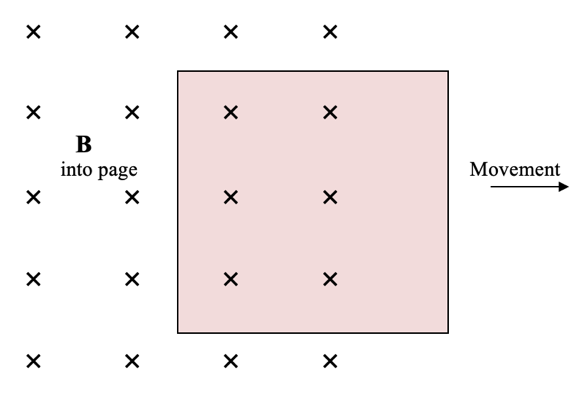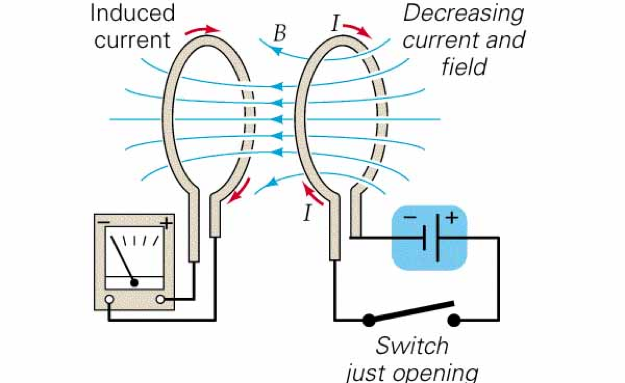# Lenz's Law and the Law of Conservation of Energy

This is part of the HSC Physics course under the topic Electromagnetic Induction.

### HSC Physics Syllabus

• analyse qualitatively and quantitatively, with reference to energy transfers and transformations, examples of Faraday's Law and Lenz's Law \varepsilon = -N (∆\phi)/(∆t), including but not limited to: (ACSPH081, ACSPH110)
– the generation of an electromotive force (emf) and evidence for Lenz's Law produced by the relative movement between a magnet, straight conductors, metal plates and solenoids
– the generation of an emf produced by the relative movement or changes in current in one solenoid int he vicinity of another solenoid

### Lenz's Law and The Law of Conservation of Energy

This video will analyse qualitatively and quantitatively, with reference to energy transfers and transformations, examples of Lenz's Law. It will also explain how it factors into the equation \varepsilon = -N (∆\phi)/(∆t).

### What is Lenz's Law?

Lenz’s Law is comes hand in hand with Faraday's Law. It states that the direction of the induced current is such that the magnetic flux produced will oppose the change in flux which induced in the emf. Consequently, the induced current's own magnetic field will interacts with the external magnetic field so as to produce a 'resisting' force.

Note: Lenz's Law is only relevant if a current is produced. That is, if an emf is induced in an open circuit where no current will flow, Lenz's Law is not applicable and a resisting force will not be felt.

### How Does the Law of Conservation of Energy Justify Lenz's Law?

The induced emf and current represents formation of electrical energy. This energy must have originated from somewhere due to Law of Conservation of Energy. That is, the kinetic energy of the relative motion between a conductor and external magnetic field must have decreased and been transformed into electrical energy. This is why it makes sense for there to be a resisting force, slowing down the relative motion.

An alternative justification is to consider a counter-example. Let us assume Lenz's Law was not true and that the induced current does not act to oppose the change in flux but instead aligns with it. This causes the induced current's magnetic field to interact with the external field such that an attractive force is developed. This attraction increases the relative motion, leads to more change in flux and thus induced current, which leads to more attraction and so on, until infinite energy has been produced. This clearly violates energy conservation!

### Relative Movement Between Magnet, Straight Conductors, Metal Plates and Solenoids

Relative movement between magnets and conductors means the magnetic flux experienced by the conductor changes. This leads to an induced emf. Such relative motion can be achieved by.

• moving a magnet in the vicinity of a conductor or
• moving a conductor within a non-uniform magnetic field

### Straight Conductors

When a straight conductor experiences changes in magnetic flux, a current is induced such that its accompanying magnetic field would oppose the change. The induced current would be in the direction so as to try to restore the magnetic flux it was originally experiencing.

For example, if a straight conductor is moved out of a magnetic field (decrease in flux), the induced current would produce a magnetic force (direction can be determined by right-hand rule) in the direction towards the magnetic field.

### Solenoids

Moving a permanent magnet in and out of a solenoid induces current. The induced current generates its own magnetic field which in turn is orientated such that it opposes the external magnetic field. The generation of current in the coils shown above is inevitably accompanied by magnetic field. The orientation of this new magnetic field is determined by the right-hand rule (shown below).For example, when the magnet is brought towards the solenoid, the solenoid experiences an increase in magnetic flux. As a result, a magnetic field is created to repel the incoming magnet. Therefore, its velocity is reduced.

When the magnet is brought away from the solenoid, the solenoid experiences a decrease in magnetic flux. As a result, a magnetic field is created to attract the incoming magnet. Therefore, its velocity is again reduced.

When the magnet is fully inside the core of the solenoid, no changes in magnetic flux are experienced by the solenoid. Consequently, the solenoid does not need to oppose any external magnetic fields so, no current is induced.

Some more examples are shown below.Observations:

• In a), the magnet is at rest so there is no deflection in the ammeter, so the needle of ammeter is at centre or zero position.
• In b), when the magnet is moved toward the coil, the needle of ammeter deflects in one direction.
• In c), when the magnet is held stationary at that position, the needle of ammeter returns back to zero position.
• In d), when the magnet is moved away from the coil, there is some deflection in the needle but in opposite direction.

### Metal Plates & Metal Sheets

The induced current in a metal plate as a result of Faraday and Lenz’s law is called eddy current, which are circular closed currents. Eddy currents are only generated in conductors of large surface area, usually metal plates, cylindrical pipes made of conducting material.

The direction of the induced eddy current (either clockwise or anti-clockwise) can be determined by the right-hand grip rule). Thumb points in the direction of the magnetic flux the metal plate desires to restore. The direction the four fingers curl in is the direction of the eddy current.For example, in the diagram above, a square metal plate is moved out of the magnetic field going into the page. As a result, the metal plate experiences a decrease in magnetic flux. Specifically, a decrease of flux which are going into the page. So, it desires to increase the number of magnetic fluxes going into the page in order to oppose the change (Lenz’s Law).

Thumb points into the page. Thus, fingers are curled in a clockwise manner. This is the direction of the resultant eddy current.

### Induction by Moving One Solenoid in the Vicinity of Another Solenoid

Since flowing current in a solenoid can produce a magnetic field that resembles a permanent magnet, two solenoids in close proximity can also display Faraday and Lenz’s law.For example, in the diagram above, the loop of wire on the right is connected to a battery whilst the loop on the left is only connected to a galvanometer. Closing and opening the switch in the right loop cause the left loop to experience an increase and decrease in magnetic flux respectively.

In both cases, by Faraday’s law, an induced current is detected by the ammeter. By Lenz’s law, the direction of current is different when the switch is closed compared with when it’s open. This is shown by the pointer being deflected in opposite direction in the galvanometer.

Shortly after the switch has been closed or opened, the induced current in the left loop disappears. This is because, the magnetic flux experienced by the left loop becomes constant (no change). This way of transmitting electrical energy from one conductor to another is called flux linkage.

Previous section: Faraday's Law of Induction

Next section: Transformer Equations